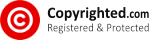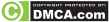• How To Calculate Cubic Metres (CBM) When ShippingDetermining Cubic Metres or CBM is the first step you should take in determining how to ship your cargo. You don’t want to be shipping FCL cargo as FCL Groupage cargo nor do you want to be shipping FCL Groupage Cargo as FCL, unless obviously you have reached or exceeded the break-even point of your cargo which I teach in the section FCL vs FCL Groupage.

The CBM of a consignment is always calculated

L x W x H
e.g. 3,20 x 2,45 x 1,56 = 12,23CBM

For Seafreight shipments, the idea is to calculate CBM in metres. If you have 320cm’s then convert it to 3,2metres before starting the calculation.

If you have 45cm’s then your formula will have 0,45metres.

A 20ft General Purpose or dry container will pack a maximum of 33,2CBM whilst a 40ft General Purpose or dry container will pack 67,7CBM.

A 40ft High Cube which will allow you more space to pack and will pack a maximum of 76,3CBM.

All of the above are subject to your line of commodity and how it is packed.

40ft General Purpose containers and High Cube containers are only to be used for larger volume cargo and not larger weight cargo. In my article on cargo weights and road weight limitations, I discuss this point further.

Also to bear in mind is the length of your cargo, you can’t pack 13 metre steel pipes in a 40ft General Purpose container as it is only 12,036metres in length. The proper equipment will be required in this case.

For airfreight shipments, the method that the airline industry uses is the Volume / Weight.

If you have 5 boxes that identical in size that are 23cmx34cmx56cm (LxBxH)
Presume that the actual weight of each of the 5 boxes is 10kgs.

What you will have to do first is separate the Volumetric from the Actual Weight.

Volumetric Weight                          |  Actual Weight

(23x34x56) div 6000                      |  10kgs per box
= 43792 div 6000
= 7,30kgs per box

For 5 boxes : 7,30 x 5 = 36,5 kgs  ( Volumetric Weight )
For 5 boxes : 10 x 5 =50 kgs ( Actual Weight )

The true weight needed to be declared to the airline here will be the actual weight of the boxes of 50kgs.

Note: Airfreight is calculated in cm’s not in metres as in seafreight.

Volumetric weight must be calculated by dividing by a denominator of 6000 in your formula when calculating cubic centimetres (cm) or dividing by 166 when calculating cubic inches (ins)

———
Article Source: http://EzineArticles.com/1041356
Re-posted by Aryaputra PandeBIC